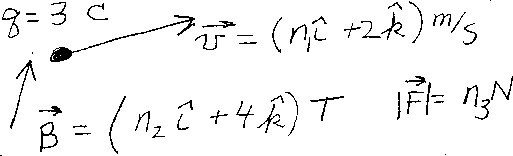Problem C11: A small object has a net charge of 3 Coulombs. At a particular time, it has a velocity v equal to v=n1 i + 2 k m/s. If it is moving in a uniform magnetic field equal to B = n2 i + 4 k Tesla, what is the magnitude of the force on the object at this time. That is, if |F| = n3 Newtons, what is n3?n1 = n2 = Input n3:
If you are currently in my class, you can record your grade by entering your name and student ID number (without the leading zeros) below and clicking on "record grade".
 First Name = Last Name = ID = Problem: